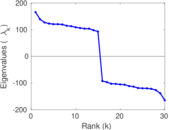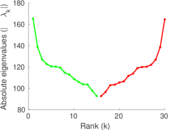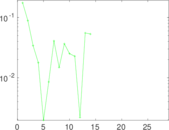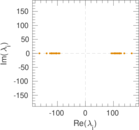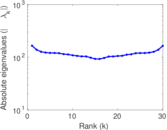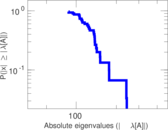This network consists of the wikilinks of the Wikipedia in the Cebuano language (ceb). Nodes are Wikipedia articles, and directed edges are wikilinks, i.e., hyperlinks within one wiki. In the wiki source, these are indicated with [[double brackets]]. Only pages in the article namespace are included.

 Code `Wceb` Internal name `wikipedia_link_ceb` Name Wikipedia links (ceb) Data source http://dumps.wikimedia.org/ AvailabilityDataset is available for download Consistency checkDataset passed all tests Category Hyperlink network Node meaning Article Edge meaning Wikilink Network format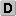Unipartite, directed Edge typeUnweighted, no multiple edges ReciprocalContains reciprocal edges Directed cyclesContains directed cycles Loops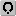Contains loops

## Statistics

 Size n = 7,891,015 Volume m = 69,540,354 Loop count l = 210 Wedge count s = 20,916,894,848,411 Claw count z = 1.474 15 × 1019 Cross count x = 1.059 2 × 1025 Triangle count t = 125,527,520 Maximum degree dmax = 3,636,006 Maximum outdegree d+max = 27,266 Maximum indegree d−max = 3,635,996 Average degree d = 17.625 2 Fill p = 1.116 79 × 10−6 Size of LCC N = 7,890,986 Size of LSCC Ns = 4,474,538 Relative size of LSCC Nrs = 0.567 042 Diameter δ = 9 50-Percentile effective diameter δ0.5 = 2.828 20 90-Percentile effective diameter δ0.9 = 4.657 40 Median distance δM = 3 Mean distance δm = 3.453 07 Balanced inequality ratio P = 0.278 849 Outdegree balanced inequality ratio P+ = 0.359 232 Indegree balanced inequality ratio P− = 0.108 641 Degree assortativity ρ = −0.189 863 Degree assortativity p-value pρ = 0.000 00 Clustering coefficient c = 1.800 38 × 10−5 Directed clustering coefficient c± = 0.018 349 6 Spectral norm α = 3,149.05 Operator 2-norm ν = 3,144.47 Reciprocity y = 0.176 973 Non-bipartivity bA = 0.002 288 73 Normalized non-bipartivity bN = 0.028 738 6

## Plots

### Degree distribution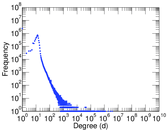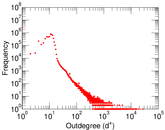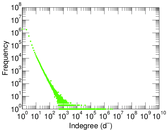### Cumulative degree distribution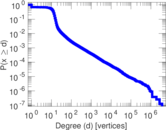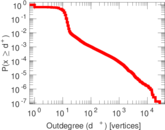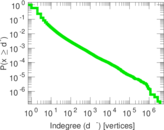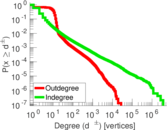### Hop distribution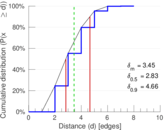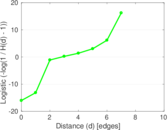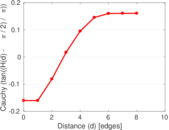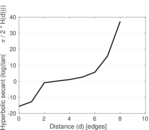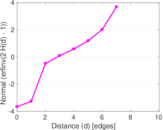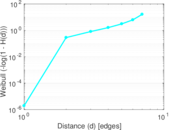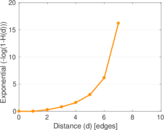### In/outdegree scatter plot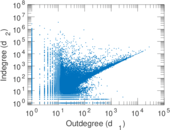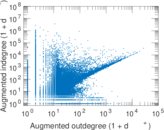### Matrix decompositions plots